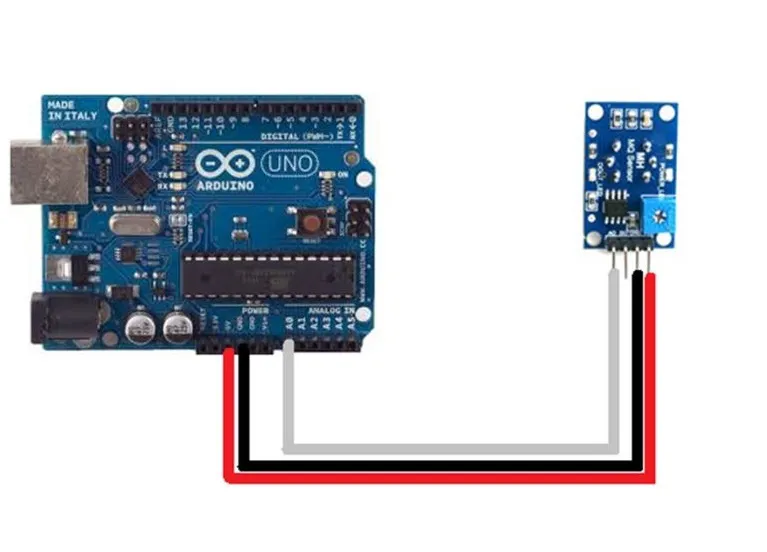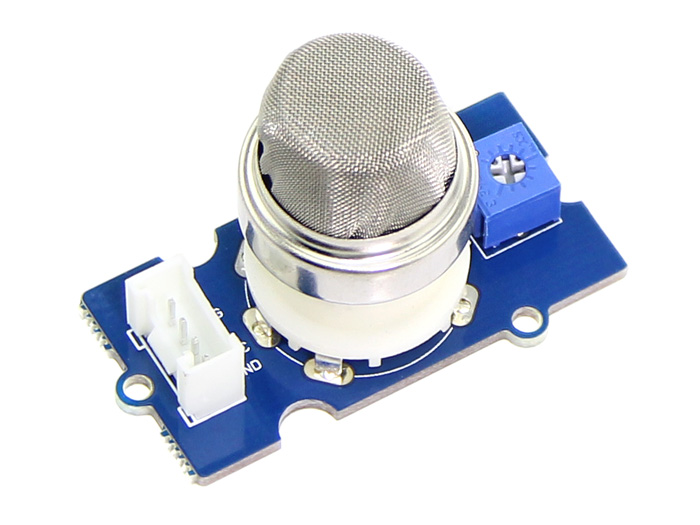Project showcase# Arduino And MQ2 Gas Sensor © CC0

The Grove Gas Sensor (MQ2) module is useful for gas leakage detection (home and industry). It is suitable for detecting H2, LPG, CH4 and CO.

• 25,150 views
• 24 respects

Video

## Code

##### Arduino CodeArduino
```#define         MQ_PIN                       (0)     //define which analog input channel you are going to use
#define         RL_VALUE                     (5)     //define the load resistance on the board, in kilo ohms
#define         RO_CLEAN_AIR_FACTOR          (9.83)  //RO_CLEAR_AIR_FACTOR=(Sensor resistance in clean air)/RO,
//which is derived from the chart in datasheet

/**********************Software Related Macros***********************************/
#define         CALIBARAION_SAMPLE_TIMES     (50)    //define how many samples you are going to take in the calibration phase
#define         CALIBRATION_SAMPLE_INTERVAL  (500)   //define the time interal(in milisecond) between each samples in the
//cablibration phase
#define         READ_SAMPLE_INTERVAL         (50)    //define how many samples you are going to take in normal operation
#define         READ_SAMPLE_TIMES            (5)     //define the time interal(in milisecond) between each samples in
#include <LiquidCrystal.h>

const int rs = 12, en = 11, d4 = 5, d5 = 4, d6 = 3, d7 = 2;
LiquidCrystal lcd(rs, en, d4, d5, d6, d7);                                                     //normal operation

/*********************Application Related Macros*********************************/
#define         GAS_LPG                      (0)
#define         GAS_CO                       (1)
#define         GAS_SMOKE                    (2)

/****************************Globals**********************************************/
float           LPGCurve  =  {2.3,0.21,-0.47};   //two points are taken from the curve.
//with these two points, a line is formed which is "approximately equivalent"
//to the original curve.
//data format:{ x, y, slope}; point1: (lg200, 0.21), point2: (lg10000, -0.59)
float           COCurve  =  {2.3,0.72,-0.34};    //two points are taken from the curve.
//with these two points, a line is formed which is "approximately equivalent"
//to the original curve.
//data format:{ x, y, slope}; point1: (lg200, 0.72), point2: (lg10000,  0.15)
float           SmokeCurve ={2.3,0.53,-0.44};    //two points are taken from the curve.
//with these two points, a line is formed which is "approximately equivalent"
//to the original curve.
//data format:{ x, y, slope}; point1: (lg200, 0.53), point2: (lg10000,  -0.22)
float           Ro           =  10;                 //Ro is initialized to 10 kilo ohms

void setup()
{
Serial.begin(9600);                               //UART setup, baudrate = 9600bps
Serial.print("Calibrating...\n");
Ro = MQCalibration(MQ_PIN);                       //Calibrating the sensor. Please make sure the sensor is in clean air
lcd.begin(16, 2);                                                  //when you perform the calibration
Serial.print("Calibration is done...\n");
Serial.print("Ro=");
Serial.print(Ro);
Serial.print("kohm");
Serial.print("\n");
lcd.print("Calibration is done...\n");
lcd.print("Ro=");
lcd.print(Ro);
lcd.print("kohm");
lcd.print("\n");
}

void loop()
{
Serial.print("LPG:");
Serial.print( "ppm" );
Serial.print("    ");
Serial.print("CO:");
Serial.print( "ppm" );
Serial.print("    ");
Serial.print("SMOKE:");
Serial.print( "ppm" );
Serial.print("\n");
lcd.setCursor(0, 0);
lcd.print("LPG:");
//lcd.print( "ppm" );
lcd.print("     ");
lcd.setCursor(9, 0);
lcd.print("CO:");
//lcd.print( "ppm" );
lcd.print("       ");
lcd.setCursor(0, 1);
lcd.print("SMOKE:");
//lcd.print( "ppm" );
lcd.print("         ");
delay(200);
}

/**************** MQResistanceCalculation **************************************
Output:  the calculated sensor resistance
Remarks: The sensor and the load resistor forms a voltage divider. Given the voltage
across the load resistor and its resistance, the resistance of the sensor
could be derived.
**********************************************************************************/
{
}

/*************************** MQCalibration **************************************
Input:   mq_pin - analog channel
Output:  Ro of the sensor
Remarks: This function assumes that the sensor is in clean air. It use
MQResistanceCalculation to calculates the sensor resistance in clean air
and then divides it with RO_CLEAN_AIR_FACTOR. RO_CLEAN_AIR_FACTOR is about
10, which differs slightly between different sensors.
**********************************************************************************/
float MQCalibration(int mq_pin)
{
int i;
float val=0;

for (i=0;i<CALIBARAION_SAMPLE_TIMES;i++) {            //take multiple samples
delay(CALIBRATION_SAMPLE_INTERVAL);
}
val = val/CALIBARAION_SAMPLE_TIMES;                   //calculate the average value

val = val/RO_CLEAN_AIR_FACTOR;                        //divided by RO_CLEAN_AIR_FACTOR yields the Ro
//according to the chart in the datasheet

return val;
}
Input:   mq_pin - analog channel
Output:  Rs of the sensor
Remarks: This function use MQResistanceCalculation to caculate the sensor resistenc (Rs).
The Rs changes as the sensor is in the different consentration of the target
gas. The sample times and the time interval between samples could be configured
by changing the definition of the macros.
**********************************************************************************/
{
int i;
float rs=0;

}

return rs;
}

/***************************  MQGetGasPercentage ********************************
Input:   rs_ro_ratio - Rs divided by Ro
gas_id      - target gas type
Output:  ppm of the target gas
Remarks: This function passes different curves to the MQGetPercentage function which
calculates the ppm (parts per million) of the target gas.
**********************************************************************************/
int MQGetGasPercentage(float rs_ro_ratio, int gas_id)
{
if ( gas_id == GAS_LPG ) {
return MQGetPercentage(rs_ro_ratio,LPGCurve);
} else if ( gas_id == GAS_CO ) {
return MQGetPercentage(rs_ro_ratio,COCurve);
} else if ( gas_id == GAS_SMOKE ) {
return MQGetPercentage(rs_ro_ratio,SmokeCurve);
}

return 0;
}

/***************************  MQGetPercentage ********************************
Input:   rs_ro_ratio - Rs divided by Ro
pcurve      - pointer to the curve of the target gas
Output:  ppm of the target gas
Remarks: By using the slope and a point of the line. The x(logarithmic value of ppm)
of the line could be derived if y(rs_ro_ratio) is provided. As it is a
logarithmic coordinate, power of 10 is used to convert the result to non-logarithmic
value.
**********************************************************************************/
int  MQGetPercentage(float rs_ro_ratio, float *pcurve)
{
return (pow(10,( ((log(rs_ro_ratio)-pcurve)/pcurve) + pcurve)));
}
```

## Schematics#### Smoke Detection using MQ-2 Gas Sensor

• 441,185 views
• 261 respects

• 44,372 views
• 15 respects

#### DHT11 Humidity + Temperature Sensor with 16x2 LCD display

Project showcase by onatto22

• 53,183 views
• 58 respects

• 29,752 views
• 27 respects

#### MQ-4 Natural Gas Sensor Experiment Based on Arduino

Project tutorial by Andrew85

• 12,840 views